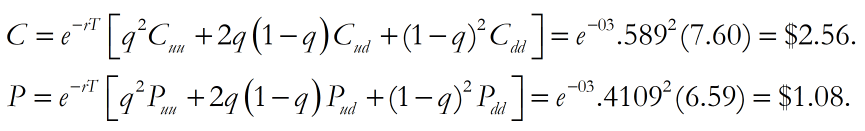# Binomial spreadsheet – replicating portfolio and risk neutral valuation approaches

Linked below is the spreadsheet we used in class today. After looking it over a bit closer, it is in fact correctly coded. My “error” was in forgetting that I had coded the 1 and 2 timestep call and put models contained therein using backward induction. Under backward induction, one calculates option prices by starting at the end of the binomial tree and working back, node by node, to the beginning of the binomial tree. Although backward induction is required to price options under the delta hedging and replicating portfolio approaches, it is not needed under the risk-neutral valuation approach.

If you open the binomial option spreadsheet, you’ll notice that the 1-timestep call and put prices obtained via backward induction are \$3.20 and \$1.72 respectively, whereas the 2-timestep call and put prices are \$2.56 and \$1.08. Of course, these are also the prices obtained via the application of the Cox-Ross-Rubinstein model equations:

1-timestep:2-timesteps:1 and 2 timestep binomial option pricing spreadsheet